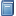Buchempfehlung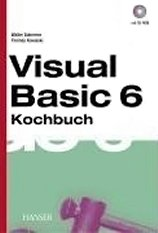Visual Basic 6 Kochbuch
Viele praktische Tipps zum Programmieren mit Visual Basic 6, die sich oft auch auf FB übertragen lassen. [Mehr Infos...]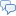FreeBASIC-Chat
Es sind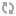Benutzer im FreeBASIC-Chat online.
(Stand:  )

# fb:porticula NoPaste

## Eine virtuelle Maschine

 Uploader: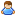AndT2008 Datum/Zeit: 08.03.2017 19:26:05

```' Das hier ist nur eine virtuelle Maschiene - enthält ein kleines Betriebsystem mit eineme Kernel und son zeug viel spass damit herauszufinden wie das funktioniert... xD #include "windows.bi" screen 0 width 80,10 color 15 locate ,,0 'settings '#define enable_bytemaker '#define boot_debugger #define allow_cls '#define sleeper '#define programmingmode #define buildmode '#define enable_debugger ' -> I USED THIS TO FIND BUGS, BUT IT CAN DEBUG DIRECTLY. I WILL GIVE NO INFORMATION TO USE THIS. <- #ifdef enable_debugger declare sub debug_vm Width 120,80 #define boot_debugger #endif dim shared exit_debugmodeflag as ushort #ifdef buildmode dim shared as ushort ram(810) dim shared as ushort r1,r2 dim shared as ubyte sram(1574) dim shared as uinteger prgctr,exitflag,nextstradr,callretadr,calladr,raster_size=16 #else dim shared as ushort ram(10024) dim shared as ubyte r1,r2 dim shared as uinteger prgctr,exitflag,nextstradr,callretadr,calladr,raster_size=16 dim shared as ubyte sram(10024) #endif dim shared as uinteger ram_checksum,sram_checksum function generate_checksum_ram (arrey() as ushort) as integer     dim as integer tmp     for i as integer = lbound(arrey) to ubound(arrey)         tmp+=i*arrey(i)     next     return tmp end function function generate_checksum_sram (arrey() as ubyte) as integer     dim as integer tmp     for i as integer = lbound(arrey) to ubound(arrey)         tmp+=i*arrey(i)     next     return tmp end function function check_ram (arrey_ram() as ushort,arrey_sram() as ubyte) as integer     if generate_checksum_ram(arrey_ram()) = ram_checksum and generate_checksum_sram(arrey_sram())= sram_checksum and ram_checksum > 0 then return 1 else return 0 end function dim shared as string u_str_input,vm_str_input function encode_string(s as string) as string     static as ubyte z     dim as string p = space(len(s))     for i as integer = 0 to len(s)-1         p[i] = s[i] xor 9876554432 + i         z+=2     next     return p end function sub compile_message_special (title as string,message as string,iconinfo as integer,targetvalue as integer,ifnotadr as integer)     title = encode_string(title)     message = encode_string(message)     ram(prgctr) = 10     ram(prgctr+1)=len(title)     ram(prgctr+2)=len(message)     ram(prgctr+3)=nextstradr     ram(prgctr+4)=iconinfo     ram(prgctr+5)=targetvalue     ram(prgctr+6)=ifnotadr     dim as integer ctr     for i as integer = nextstradr to nextstradr+ram(prgctr+1)-1         sram(i)=title[ctr]         ctr+=1     next     nextstradr = nextstradr+ram(prgctr+1)     ctr = 0     for i as integer = nextstradr to nextstradr+ram(prgctr+2)-1         sram(i)=message[ctr]         ctr+=1     next     nextstradr = nextstradr+ram(prgctr+2)     prgctr+=7 end sub sub compile_message (title as string,message as string,iconinfo as integer)     title = encode_string(title)     message = encode_string(message)     ram(prgctr) = 1     ram(prgctr+1)=len(title)     ram(prgctr+2)=len(message)     ram(prgctr+3)=nextstradr     ram(prgctr+4)=iconinfo     dim as integer ctr     for i as integer = nextstradr to nextstradr+ram(prgctr+1)-1         if i > ubound(sram) then exit sub         sram(i)=title[ctr]         ctr+=1     next     nextstradr = nextstradr+ram(prgctr+1)     ctr = 0     for i as integer = nextstradr to nextstradr+ram(prgctr+2)-1         sram(i)=message[ctr]         ctr+=1     next     nextstradr = nextstradr+ram(prgctr+2)     prgctr+=5 end sub sub set_r2(value as integer)     ram(prgctr)=2     ram(prgctr+1)=value     prgctr+=2 end sub sub compile_jump(value as integer)     ram(prgctr)=3     ram(prgctr+1)=value     prgctr+=2 end sub sub compile_check(if_yes_adr as integer)     ram(prgctr)=4     ram(prgctr+1)=if_yes_adr     prgctr+=2 end sub sub compile_exit     'print "EXIT ON :";prgctr     ram(prgctr)=5     prgctr+=1 end sub sub compile_set_r2(value as integer)     ram(prgctr)=6     ram(prgctr+1)=value     prgctr+=2 end sub sub compile_stop_app     compile_message ("Hidden security function","Nobody can crack this.. Retry?",MB_YESNO)     compile_check 0     compile_message ("NOOB!","GIVE UP... :D",MB_OK)     prgctr = 15     compile_message ("Hahaha","Come on you can't do this :)",MB_ICONINFORMATION)     compile_exit end sub sub secure_app     ram(prgctr)=7     prgctr+=1 end sub sub check_r2(value as integer,jumpadr as integer)     ram(prgctr)=8     ram(prgctr+1)=value     ram(prgctr+2)=jumpadr     prgctr+=3 end sub sub check_r2_inv(value as integer,jumpadr as integer)     ram(prgctr)=9     ram(prgctr+1)=value     ram(prgctr+2)=jumpadr     prgctr+=3 end sub sub compile_sleep(value as ushort)     ram(prgctr)=28     ram(prgctr+1)=value     prgctr+=2 end sub randomize timer sub run_vm do        ' ram(int(rnd*ubound(ram)))=int(rnd*256)     #ifdef boot_debugger '    boot debugger     dim as ubyte h_line = csrlin     static as ubyte z_line = 24     z_line+=1     if z_line > 50 then z_line = 24     locate z_line,1     print prgctr,ram(prgctr),r1,r2,callretadr,calladr;"  "     locate h_line     #endif     #ifdef sleeper         sleep 15,1     #endif     if prgctr < lbound(ram) then prgctr = 0     if prgctr > ubound(ram) then compile_message ("ERROR!","Rom is to strong corrupted (prgctr="+str(prgctr)+")",MB_ICONERROR) : prgctr = 0 : exitflag = 1     'print "PRGCTR ";prgctr     select case ram(prgctr)     case 1         randomize timer         'PRINT "MESSAGE"         dim as integer titlelength = ram(prgctr+1)         dim as integer messagelength = ram(prgctr+2)         dim as integer titleadr = ram(prgctr+3)         dim as integer iconinfo = ram(prgctr+4)         dim as integer messageadr = titleadr+titlelength         dim as string title,message         for i as integer = titleadr to titleadr+titlelength - 1             title+= chr(sram(i))         next         for i as integer = messageadr to messageadr+messagelength - 1             message+= chr(sram(i))         next         title = encode_string(title)         message =encode_string(message)         r1 = MessageBox(0,message,title,iconinfo)      prgctr+=4  case 2      'print "R2 IS ";R2      r2 = ram(prgctr+1)      'print "SET R2 TO ";r2      prgctr+=1  case 3      'print "JUMP FROM ";prgctr;" TO ";ram(prgctr+1)     prgctr=ram(prgctr+1)-1  case 4      'print "R1:";R1      'print "V:";IDYES      if r1 = IDYES then      '    print "OLDPTR: ";prgctr          'print "VALUE :";ram(prgctr+1)          prgctr = ram(prgctr+1)-1          'print "NEWPTR:";prgctr      else          prgctr+=1      end if  case 5      exitflag = 1  case 6      r2 = ram(prgctr+1)      prgctr+=1     case 7          erase(ram)         compile_stop_app     case 8         if r2 = ram(prgctr+1) then             prgctr = ram(prgctr+2)-1         else             prgctr+=2         end if     case 9         if r2 <> ram(prgctr+1) then             prgctr = ram(prgctr+2)-1         else             prgctr+=2         end if      case 10         dim as uinteger titlelength = ram(prgctr+1)         dim as uinteger messagelength = ram(prgctr+2)         dim as uinteger titleadr = ram(prgctr+3)         dim as uinteger iconinfo = ram(prgctr+4)         dim as uinteger ifnotadr = ram(prgctr+6)         dim as uinteger messageadr = titleadr+titlelength         dim as string title,message         for i as integer = titleadr to titleadr+titlelength - 1             title+= chr(sram(i))         next         for i as integer = messageadr to messageadr+messagelength - 1             message+= chr(sram(i))         next         if r2 = ram(prgctr+5) then             title = encode_string(title)             message =encode_string(message)             r1 = MessageBox(0,message,title,iconinfo)         else             'print "OLDADR :";prgctr             prgctr = ifnotadr-1             'print ifnotadr             'print "NEWADR :";prgctr         end if      prgctr+=6  case 11      'print "INC 1"      r2+=1      'print "R2 = ",r2  case 12      'print "INC 10"      r2+=10  case 13      'print "INC 100"      r2+=100     case 14 'call         'print "CALL ";         calladr = ram(prgctr+1)-1         'print calladr +1         callretadr = prgctr         prgctr = calladr     case 15 'returncall         'print "RETURN"         if callretadr = 0 then print "Return without call@";prgctr : exit select         prgctr = callretadr+1         callretadr = 0     case 16      'print "CHECKCALL"      'print "R1:";R1      'print "V:";IDYES      if r1 = IDYES then          calladr = ram(prgctr+1)-1          callretadr = prgctr          prgctr = calladr      else          prgctr+=1      end if  case 17 'debugmsg     dim as string message     dim as uinteger length = ram(prgctr+1)     dim as uinteger msgtyp = ram(prgctr+3)     dim as uinteger msgadress = ram(prgctr+2)     for i as integer = msgadress to msgadress + length -1         message +=chr(sram(i))     next     message = encode_string(message)     color 15     print "DEBUG: ";     color 7     select case msgtyp     case 0         print message     case 1         color 15         print "[INFO] [";         print message;"]"         color 7     case 2         color 14         print "[WARNING] [";         print message;"]"         color 7      case 3          color 12          print "[ERROR] [";          print message;"]"          color 7     case else         print "[INVAILED MSGTYP]@ ";prgctr;"[";         print message;"]"     end select     prgctr+=3  case 18      'print "call if r2"      'print "R2 =";r2     if r2 = ram(prgctr+1) then         'print "true"          calladr = ram(prgctr+2)-1          callretadr = prgctr + 1          prgctr = calladr      else          'print "false"          prgctr+=2      end if   case 19       u_str_input = ""       dim as string message     dim as uinteger length = ram(prgctr+1)     dim as uinteger hidemode = ram(prgctr+3)     dim as uinteger msgadress = ram(prgctr+2)     for i as integer = msgadress to msgadress + length -1         message +=chr(sram(i))     next     message = encode_string(message)     print message;     if hidemode = 0 then         input "->",u_str_input     else         dim as ubyte char         print "->";         do             char = asc(inkey)             if char = 13 then exit do             if char > 0 then                 print "*";                 u_str_input+=chr(char)             end if         loop     end if     prgctr+=3    case 20     dim as string message     dim as uinteger length = ram(prgctr+1)     dim as uinteger msgtyp = ram(prgctr+3)     dim as uinteger msgadress = ram(prgctr+2)     for i as integer = msgadress to msgadress + length -1         message +=chr(sram(i))     next     message = encode_string(message)     vm_str_input = message     prgctr+=3 case 21     if u_str_input = vm_str_input then         r2 = 1     else         'print u_str_input,vm_str_input         r2 = 0     end if case 22     dim as string message     dim as uinteger length = ram(prgctr+1)     dim as uinteger msgadress = ram(prgctr+2)     for i as integer = msgadress to msgadress + length -1         message +=chr(sram(i))     next     message = encode_string(message)     print message     prgctr+=2 case 23     locate ram(prgctr+1),ram(prgctr+2)     prgctr +=2 case 24     beep     locate csrlin+1,ram(prgctr+1)     prgctr+=1 case 25     #ifdef allow_cls     cls     #endif case 26     color ram(prgctr+1),ram(prgctr+2)     prgctr+=2 case 27     r2 = check_ram(ram(),sram()) case 28     sleep ram(prgctr+1)     prgctr+=1 #ifdef enable_debugger case 29     prgctr = 0     debug_vm #endif case 30     screenres 1280,768 case 31     dim as ushort x,y,c     x = ram(prgctr+1)     y = ram(prgctr+2)     c = ram(prgctr+3)     line(x * raster_size, y * raster_size) - ((x*raster_size)+raster_size,(y*raster_size)+raster_size),15,bf     prgctr+=3 case 32     raster_size = ram(prgctr+1)     prgctr+=1 case 33     screen 0 case else      print "INVAILED OPCODE";ram(prgctr);"@";prgctr  end select  prgctr+=1 loop until exitflag = 1 end sub sub compile_openscreen     ram(prgctr)=30     prgctr+=1 end sub sub compile_closescreen     ram(prgctr)=33     prgctr+=1 end sub sub compile_set_pixel (x as ushort,y as ushort,c as ushort)     ram(prgctr)=31     ram(prgctr+1)=x     ram(prgctr+2)=y     ram(prgctr+3)=c     prgctr+=4 end sub sub compile_H (x as integer,y as integer)     for i as integer = 0 to 4         compile_set_pixel x,y+i,15         compile_set_pixel x+3,y+i,15     next     for i as integer = 0 to 1         compile_set_pixel x+i+1,y+2,15     next end sub sub compile_E(x as integer,y as integer)     for i as integer = 0 to 4         compile_set_pixel x,y+i,15     next     for i as integer = 1 to 2         compile_set_pixel x+i,y,15         compile_set_pixel x+i,y+2,15         compile_set_pixel x+i,y+4,15     next end sub sub compile_L (x as integer,y as integer)     for i as integer = 0 to 4         compile_set_pixel x,y+i,15     next     for i as integer = 1 to 2         compile_set_pixel x+i,y+4,15     next end sub sub compile_O(x as integer,y as integer)     for i as integer = 1 to 2         compile_set_pixel x+1+i,y,15         compile_set_pixel x+i+1,y+5,15     next     for i as integer = 1 to 4         compile_set_pixel x+1,y+i,15         compile_set_pixel x+4,y+i,15     next end sub 'for i as integer = sub update(adr as ushort,value as ushort)     ram(adr)=value end sub #ifdef enable_debugger sub modify     do         dim as short adr,value         input "ADRESS :";adr         select case adr         case -1             exit_debugmodeflag = 1             exit sub         case -2             exit_debugmodeflag = 1             run_vm             exit sub         case -3             exit_debugmodeflag = 1             print "PRGCTR ->";             input "",prgctr             prgctr-=1             cls             run_vm             exit sub         case -4             exit do         end select         input "VALUE  :";value         update adr,value         prgctr = 0     loop end sub sub debug_vm     windowtitle "Debugger"     dim as ubyte char     do         if exit_debugmodeflag = 1 then             exit_debugmodeflag = 0             exit do         end if         print "ADR :";prgctr ;" ";     select case ram(prgctr)     case 1         print "MESSAGE";         dim as integer titlelength = ram(prgctr+1)         dim as integer messagelength = ram(prgctr+2)         dim as integer titleadr = ram(prgctr+3)         dim as integer iconinfo = ram(prgctr+4)         dim as integer messageadr = titleadr+titlelength         dim as string message         print "TLENGTH = ";titlelength;",";         print "MLENGTH =";messagelength;",";         print "TADR =";titleadr;",";         print "II = ";iconinfo;",";         print "MADR = ";messageadr;",";         for i as integer = messageadr to messageadr + messagelength -1             message +=chr(sram(i))         next         message = encode_string(message)         print "MESSAGE -> "; message         prgctr+=4     case 2         print "SET R2, VALUE = ";ram(prgctr+1)         prgctr+=1     case 3         print "GOTO ";ram(prgctr+1)         prgctr+=1     case 4         print "YES BUTTON CHECK, GOTO ADR = ";ram(prgctr+1)         prgctr+=1     case 5         PRINT "SET EXITFLAG"     case 6         PRINT "SET R2, VALUE = ";ram(prgctr+1)         prgctr+=1     case 7         PRINT "ERASE RAM"     case 8         PRINT "IF R2 = ";ram(prgctr+1); " GOTO ";ram(prgctr+2)         prgctr+=2     case 9         PRINT "IF R2 <> ";ram(prgctr+1); " GOTO ";ram(prgctr+2)         prgctr+=2     case 10         PRINT "MESSAGE SPECIAL"          dim as uinteger titlelength = ram(prgctr+1)         dim as uinteger messagelength = ram(prgctr+2)         dim as uinteger titleadr = ram(prgctr+3)         dim as uinteger iconinfo = ram(prgctr+4)         dim as uinteger ifnotadr = ram(prgctr+6)         dim as uinteger messageadr = titleadr+titlelength          print "         TLENGTH = ";titlelength;",";         print "MLENGTH =";messagelength;",";         print "TADR =";titleadr;",";         print "II = ";iconinfo;",";         print "MADR = ";messageadr;         print "R2TVAL = ";ram(prgctr+6)         print         prgctr+=6     case 11         PRINT "R2 + = 1"     case 12         PRINT "R2 + = 10"     case 13         print "R2 + = 100"     case 14         print "CALL, ADR =";ram(prgctr+1)         prgctr+=1     case 15         print "RETURN"     case 16         print "IF BUTTON = YES THEN CALL ";ram(prgctr+1)         prgctr+=1     case 17          dim as string message     dim as uinteger length = ram(prgctr+1)     dim as uinteger msgtyp = ram(prgctr+3)     dim as uinteger msgadress = ram(prgctr+2)     for i as integer = msgadress to msgadress + length -1         message +=chr(sram(i))     next     message = encode_string(message)         print "DEBUGMSG,";         print "TYPE = ";         select case ram(prgctr+3)         case 0             color 15         print "[NULL]";         color 7     case 1         color 15         print "[INFO]";         color 7     case 2         color 14         print "[WARNING]";'         color 7      case 3          color 12          print "[ERROR]";'' [";          color 7     case else         print "[UNKNOWN MSGTYP]";     end select     color 15     print "->";message     color 7     prgctr+=3     case 18         print "CALL IF R2, R2 TARGETVALUE =";ram(prgctr+1);", CALLADR =" ;ram(prgctr+2)         prgctr+=2     case 19         print "USERINPUT, TYPE :";ram(prgctr+3)         prgctr+=3     case 20         print "READ VM STRING"         prgctr+=3     case 21         print "COMPARE STRINGS"     case 22         print "SIMPLE STRING OUTPUT"         prgctr+=2     case 23         PRINT "LOCATE ";ram(prgctr+1);",";ram(prgctr+2)         prgctr+=2     case 24         print "LOCATE WITH NEW LINE ",ram(PRGCTR+1)         prgctr+=1     case 25         print "CLEAR SCREEN"     case 26         PRINT "COLOR,", ram(prgctr+1);",";ram(prgctr+2)         prgctr+=2     case 27         print "CHECK ROM"     case 28         print "SLEEP ";ram(prgctr+1)         prgctr+=1             case 29     print "RUN DEBUG VM"      case 30     Print "OPENSCREEN" case 31          dim as ushort x,y,c     x = ram(prgctr+1)     y = ram(prgctr+2)     c = ram(prgctr+3)     PRINT "SETPIXEL ->";x;" ";y;" ";c     prgctr+=3 case 32     PRINT "SET RASTERSIZE" case 33     PRINT "SCREEN 0" case else         print "unknown opcode"     end select     prgctr+=1          if csrlin >= 70 then                 sleep         select case asc(inkey)         case 8             modify         end select                  cls         locate 1     end if     loop until prgctr >=ubound(ram) end sub sub compile_debugmode     ram(prgctr)=29     prgctr+=1 end sub #endif sub compile_color(main_col as ubyte,bg_col as ubyte)     ram(prgctr)=26     ram(prgctr+1)=main_col     ram(prgctr+2)=bg_col     prgctr+=3 end sub sub compile_cls     ram(prgctr)=25     prgctr+=1 end sub sub compile_next_line (y as ushort)     ram(prgctr)=24     ram(prgctr+1)=y     prgctr+=2 end sub sub compile_locate (x as ushort,y as ushort)     ram(prgctr)=23     ram(prgctr+1)=x     ram(prgctr+2)=y     prgctr +=3 end sub sub compile_compare_input     ram(prgctr)=21     prgctr+=1 end sub sub compile_sys_input (masg as string,msgtyp as ubyte = 0)     masg = encode_string(masg)     dim as uinteger startadress = nextstradr     dim as uinteger endadress = startadress + len(masg)     dim as uinteger ctr     if len(masg) > 0 then         for i as integer = startadress to endadress             sram(i)=masg[ctr]             ctr+=1         next     end if          ram(prgctr)=20     ram(prgctr+1)=len(masg)     ram(prgctr+2)=startadress     ram(prgctr+3)=msgtyp     prgctr+=4     nextstradr = endadress + 1 end sub sub compile_u_input (masg as string,hidemode as integer = 0)     masg = encode_string(masg)     dim as uinteger startadress = nextstradr     dim as uinteger endadress = startadress + len(masg)     dim as uinteger ctr     for i as integer = startadress to endadress         sram(i)=masg[ctr]         ctr+=1     next     ram(prgctr)=19     ram(prgctr+1)=len(masg)     ram(prgctr+2)=startadress     ram(prgctr+3)=hidemode     prgctr+=4     nextstradr = endadress + 1 end sub sub call_if_r2 (r2_val as ushort,adress as ushort)     ram(prgctr)=18     ram(prgctr+1)=r2_val     ram(prgctr+2)=adress     prgctr+=3 end sub sub compile_print(masg as string)         masg = encode_string(masg)     dim as uinteger startadress = nextstradr     dim as uinteger endadress = startadress + len(masg)     dim as uinteger ctr     for i as integer = startadress to endadress         sram(i)=masg[ctr]         ctr+=1     next     ram(prgctr)=22     ram(prgctr+1)=len(masg)     ram(prgctr+2)=startadress     prgctr+=3     nextstradr = endadress + 1 end sub sub debug_message (masg as string,msgtyp as ubyte = 0)     masg = encode_string(masg)     dim as uinteger startadress = nextstradr     dim as uinteger endadress = startadress + len(masg)     dim as uinteger ctr     for i as integer = startadress to endadress         sram(i)=masg[ctr]         ctr+=1     next     ram(prgctr)=17     ram(prgctr+1)=len(masg)     ram(prgctr+2)=startadress     ram(prgctr+3)=msgtyp     prgctr+=4     nextstradr = endadress + 1 end sub           sub write_rom     open "msgboxvm.rom" for binary access write as #1     put #1,,ram_checksum     put #1,,sram_checksum     put #1,,ram()     put #1,,sram()     close #1 end sub FUNCTION load_rom AS INTEGER     dim as integer result = open ("msgboxvm.rom" for binary access read as #1)     if result = 0 then         get #1,,ram_checksum         get #1,,sram_checksum         get #1,,ram()         get #1,,sram()         close #1     end if     return result end function sub compile_inc_r2_1     ram(prgctr)=11     prgctr+=1 end sub sub compile_inc_r2_10     ram(prgctr)=12     prgctr+=1 end sub sub compile_inc_r2_100     ram(prgctr)=13     prgctr+=1 end sub sub compile_call(calladr as ushort)     ram(prgctr)=14     ram(prgctr+1)=calladr     prgctr+=2 end sub sub compile_check_call(calladr as ushort)     ram(prgctr)=16     ram(prgctr+1)=calladr     prgctr+=2 end sub sub compile_return     ram(prgctr)=15     prgctr+=1 end sub sub c_debug (s as string = "")     color 15     print "Compile debugger -> ";S;prgctr     color 7 end sub sub compile_ask_password (password as string)     compile_u_input "Password",1     compile_sys_input password     compile_compare_input end sub sub compile_check_ram     ram(prgctr)=27     prgctr+=1 end sub      function set_placeholder as ushort     prgctr+=2     return prgctr     end function sub compile_user_input (Text as string)     compile_u_input Text,0 end sub sub compile_check_input_call (s as string,call_adr as ushort) compile_sys_input s compile_compare_input call_if_r2 1,call_adr end sub sub compile_check_input_jump (s as string,jump_adr as ushort) compile_sys_input s compile_compare_input call_if_r2 1,jump_adr end sub 'here is i programming the code for the vm '#include "buildrom.bas"compi sub compile_app 'Kernel dim as ubyte main_area = 90 dim as ushort c_calladr debug_message "Booting kernel.." c_calladr = prgctr prgctr+=2 compile_jump 234 'compile_jump main_area dim as integer msg_bad_password = prgctr compile_message "ERROR","Bad password",mb_iconerror+mb_ok dim as integer ksize = prgctr compile_exit dim as ushort exit_area = prgctr compile_message "OK","Exit is here",mb_iconinformation+mb_ok compile_exit dim as ushort repeat_quest = prgctr compile_message "A question","Repeat this?",mb_yesno+mb_iconinformation check_r2 0,main_area set_r2 10 compile_check 145 compile_return dim as ushort fatal_error_msg = prgctr debug_message "Unexepted exeption",3 compile_exit dim as ushort empty_password = prgctr compile_message "Input error!","Empty Password",mb_ok+mb_iconerror compile_exit dim as ushort msg_fail = prgctr debug_message "Kernel not initalized",3 compile_exit dim as ushort msg_kernel_load_error = prgctr debug_message "Kernel not loaded!",3 compile_exit dim as ushort msg_maschine_error = prgctr debug_message "Maschine failure detected" debug_message "REBOOT with failure flag" set_r2 128 compile_jump 0 compile_exit dim as ushort msg_main_core_error = prgctr debug_message "Main application bad!",2 compile_exit dim as ushort msg_main_error = prgctr debug_message "Kernelsize :"+str(prgctr - ksize)+"bytes" compile_return dim as ushort k_boot = prgctr debug_message "Setting up Kernel" set_r2 9 debug_message "Done",1 compile_return compile_jump msg_fail print prgctr 'MAIN AREA HERE debug_message "Calling kernel.." call_if_r2 128,msg_bad_password call_if_r2 1,k_boot 'BUG HERE! debug_message "Checking kernel state.." check_r2 0,msg_fail debug_message "Success" compile_message "Hi!","Are you ready?",MB_yesno+mb_iconinformation compile_check 145 compile_jump exit_area debug_message "Main application is glitching",2 compile_jump msg_fail compile_exit compile_call msg_maschine_error debug_message "msg yes area" dim as ubyte optr,nptr compile_check 145 compile_jump msg_main_core_error print "JEY ADREA ";PRGCTR check_r2 10,145 check_r2 9, 145 compile_jump fatal_error_msg print "JUMPADR ";prgctr 'sleep for i as integer = 0 to 3     compile_sys_input "medusa"     compile_compare_input     check_r2 0,msg_bad_password         compile_message "HI!","This is message : "+str(i),mb_ok+mb_iconinformation     compile_jump prgctr+4     compile_jump msg_main_core_error     next compile_call repeat_quest compile_jump exit_area 'run compile_print "No jump failed!" compile_jump fatal_error_msg print "LOCKKER :";Prgctr 'sleep dim as ubyte msg_not_unlocked = prgctr compile_inc_r2_1 compile_message "Message from Crackme","Crackme not unlocked, unlock crackme first.", mb_iconinformation+mb_ok check_r2_inv 2,msg_fail compile_exit compile_jump fatal_error_msg print "MAIN JUMP : ";prgctr compile_cls const anim = 31 dim as string upstr = chr(218) for i as integer = 1 to anim     upstr+=chr(196) next upstr+=chr(191) compile_print upstr compile_locate 2,1 compile_print chr(179)+SPACE(anim)+chr(179) compile_locate 2,3 compile_color 15,0 compile_print "MrMinecraft's Crackme #1 v0.1" compile_color 7,0 dim as string downstr = chr(192) for i as integer = 1 to anim     downstr+=chr(196) next downstr+=chr(217) compile_print downstr compile_locate 5,2 compile_print "You have to modify the msgboxvm.rom - good look :)" compile_check_ram 'compile_call 222 check_r2_inv 1,msg_not_unlocked compile_message "Message from Crackme","Now you have to enter the correct Activation password - good look :)",mb_iconinformation+mb_ok compile_print "Need Password to activate this application.." compile_u_input "Password",1 compile_compare_input check_r2 1,empty_password compile_sys_input "medusa" compile_compare_input check_r2 1,main_area debug_message "NOT PASSED" set_r2 128 compile_jump msg_bad_password compile_exit compile_exit dim as ushort k_looper = prgctr compile_sleep 255 compile_jump k_looper dim as ushort k_bootloop_detected = prgctr compile_locate 4,16 compile_color 12,0 compile_print "." compile_color 7,0 compile_locate 46,20 debug_message "Bootloop detected!!!",3 #ifdef enable_debugger     compile_debugmode #else compile_jump k_looper #endif dim as ushort k_start_main = prgctr compile_return dim as ushort k_check_r0_failed = prgctr debug_message "R2 NOT 0" compile_exit dim as ushort k_check_pw_check_failed = prgctr debug_message "Password check failed",3 compile_exit dim as ushort k_check_inc_r2_failed = prgctr debug_message "INC R2 is not working correctly",3 compile_exit dim as ushort k_demo = prgctr compile_openscreen compile_H 1,4 compile_E 6,4 compile_L 10,4 compile_L 13,4 compile_O 16,3 compile_sleep 1024 compile_closescreen   set_r2 9 compile_return print prgctr #ifdef enable_debugger dim as ushort k_debugger = prgctr compile_openscreen compile_debugmode compile_closescreen compile_print "DEBUGMODE LEFT" compile_return #endif dim as ushort k_info = prgctr compile_cls compile_color 15,0 compile_locate 2,2 compile_print "Hello Guys!" compile_locate 3,2 compile_print "This little Crackme is designed to crack. It is legal to do this." compile_locate 4,2 compile_print "Because the programmer (me) has allowed to do this." compile_locate 6,2 compile_print "How to Crack: " compile_print "Reverse my virtual machine and edit the msgboxvm.rom with an hexeditor." compile_locate 9,15 compile_print " And now have fun! :)" compile_user_input "Press Enter to return." set_r2 10 compile_return dim as ushort k_bad_selection = prgctr compile_print "Bad Selection." compile_return dim as ushort k_select = prgctr compile_cls compile_color 15,0 set_r2 0 compile_print "Options :" compile_print "1 -> Starting Crackme" compile_print "2 -> Info" compile_print "3 -> Exit" compile_print "4 -> Demo" #ifdef enable_debugger compile_print "5 -> Debugger" #endif compile_user_input "Select an Option " compile_check_input_jump "1",234 compile_check_input_call "2",k_info compile_check_input_jump "3",exit_area compile_check_input_jump "4",k_demo set_r2 10 #ifdef enable_debugger compile_check_input_call "5",k_debugger #endif call_if_r2 10,k_bad_selection compile_jump k_select dim as ushort targetadr = prgctr prgctr = c_calladr compile_call targetadr prgctr = targetadr compile_cls debug_message "BOOTING.." debug_message "Loading MrMinecraft's Kernel.." compile_locate 2,40 debug_message "KernelVersion -> 0.2" debug_message "INITALIZE KERNEL",1 compile_locate 4,1 debug_message "State :[....]" check_r2 128,k_bootloop_detected debug_message "Checking SYS input.." compile_sys_input "" compile_compare_input check_r2 0,k_check_pw_check_failed debug_message "Passed",1 compile_locate 4,16 compile_color 10,0 compile_print "." compile_color 7,0 compile_locate 7,1 set_r2 0 check_r2_inv 0,k_check_r0_failed debug_message "Checking Incraser functions..",0 compile_inc_r2_1 compile_inc_r2_10 compile_inc_r2_100 check_r2_inv 111,k_check_inc_r2_failed compile_locate 4,17 compile_color 10,0 compile_print "." compile_color 7,0 compile_locate 8,1 compile_color 2,0 debug_message "Set R2 to 0.." set_r2 0 compile_color 7,0 compile_locate 4,18 compile_color 10,0 compile_print "." compile_color 7,0 compile_locate 9,1 debug_message "Starting main programm..",1 compile_locate 4,19 compile_color 10,0 compile_print "." compile_color 7,0 compile_locate 10,1 compile_jump k_select ram_checksum = generate_checksum_ram(ram()) sram_checksum = generate_checksum_sram(sram()) end sub sub bytemaker     open "bytes2.bas" for output as #1     print #1,"dim shared as ushort ram(";ubound(ram);") =>{";     for i as integer = 0 to ubound(ram)-1         print #1,ram(i);         if i < ubound(ram)-1 then print #1,",";     next     print #1,"}"     print #1,"dim shared as ubyte sram(";ubound(sram);") =>{";     for i as integer = 0 to ubound(sram)-1         print #1,sram(i);         if i < ubound(sram)-1 then print #1,",";     next     print #1,"}" end sub print "LOADING ROM.."; if open ("msgvmbox.rom" for binary access read as #1) = 0 then     close #1     load_rom     PRINT "OK" else     compile_app     print "NOT FOUND - CREATE ROM.."     write_rom     PRINT "OK" end if print "RUN VM.."; prgctr = 0 run_vm```